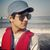# QlikView App Dev

Discussion Board for collaboration related to QlikView App Development.

Announcements
Support Case Portal has moved to Qlik Community! Read the FAQs to start exploring Support resources.
cancel
Showing results for
Did you mean:Creator

## List Box Range Values

Hi Guys,

I need your help. Actually I am trying to do display the results on the basis of List box selection.  Following is My SQL script .

CASE

when Interval BETWEEN 0 and 6 then Less then 7 days

when Interval BETWEEN '0' and 13 then Less then 14 days

when Interval BETWEEN 0 and 27 then Less then 28 days

when Interval BETWEEN 0 and 31 then Less then 30 days

The issue with the above script is it gives wrong values. Like Less then 14 Days will give you values from  7 -13 .  not  from 0-13. same like Less then 28 days  show values between 13 and 26.

My list box (please review attached picture) given me wrong data .

Is there a way I can manage in Qlikview?

Kind Regards

Waqas Shah

Labels (3)

• ### List Box Range Values

5 RepliesSpecialist
Try this :
CASE

when Interval >=0 and Interval <= 6 then Less then 7 days

when Interval >=0 and Interval <= 13 then Less then 14 days

when Interval >=0 and Interval <= 27 then Less then 28 days

when Interval >=0 and Interval <=31 then Less then 30 daysCreator
Author

Unfortunately its not working. any other option ?Specialist

write it properly,

CASE
when Interval BETWEEN 0 and 6 then 'less then 7 days' //with ( ' ) after then
when Interval BETWEEN '0'  0 and 13 then 'Less then 14 days'
when Interval BETWEEN 0 and 27 then 'Less then 28 days'
when Interval BETWEEN 0 and 31 then 'Less then 30 days'

end as xyzCreator
Author

Sorry I did not get you . you mean trying to  add (') in SQL script ?Specialist

CASE
when Interval BETWEEN 0 and 6 then less then 7 days
when Interval BETWEEN '0' and 13 then Less then 14 days
when Interval BETWEEN 0 and 27 then Less then 28 days
when Interval BETWEEN 0 and 31 then Less then 30 days

My suggestion :

CASE
when Interval BETWEEN 0 and 6 then 'less then 7 days'
when Interval BETWEEN 0 and 13 then 'Less then 14 days'
when Interval BETWEEN 0 and 27 then 'Less then 28 days'
when Interval BETWEEN 0 and 31 then 'Less then 30 days'Community Browser